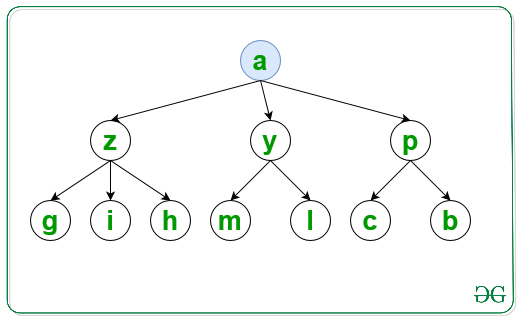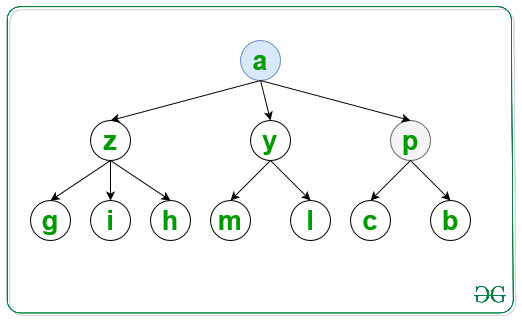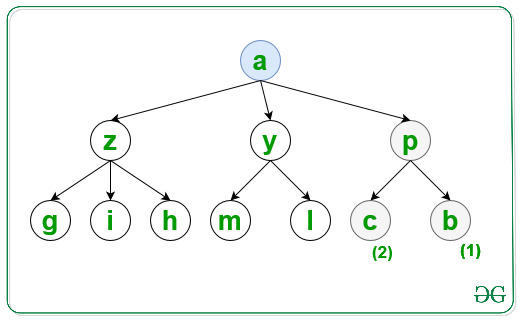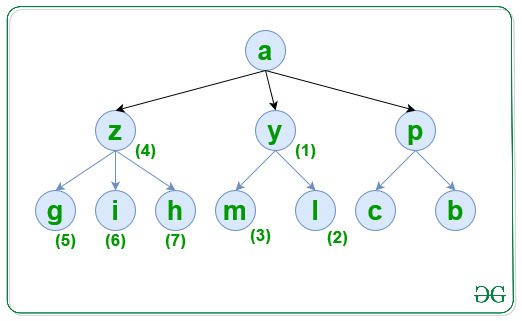Related Articles

# Traverse graph in lexicographical order of nodes using DFS

• Last Updated : 20 Jul, 2021

Given a graph, G consisting of N nodes, a source S, and an array Edges[] of type {u, v} that denotes that there is an undirected edge between node u and v, the task is to traverse the graph in lexicographical order using DFS.

Examples:

Input: N = 10, M = 10, S = ‘a’, Edges[] = { { ‘a’, ‘y’ }, { ‘a’, ‘z’ }, { ‘a’, ‘p’ }, { ‘p’, ‘c’ }, { ‘p’, ‘b’ }, { ‘y’, ‘m’ }, { ‘y’, ‘l’ }, { ‘z’, ‘h’ }, { ‘z’, ‘g’ }, { ‘z’, ‘i’ } }
Output: a p b c y l m z g h i

Explanation:
For the first level visit the node and print it:Similarly visited the second level node p which is lexicographical smallest as:Similarly visited the third level for node p in lexicographical order as:Now the final traversal is shown in the below image and labelled as increasing order of number:Input: N = 6, S = ‘a’, Edges[] = { { ‘a’, ‘e’ }, { ‘a’, ‘d’ }, { ‘e’, ‘b’ }, { ‘e’, ‘c’ }, { ‘d’, ‘f’ }, { ‘d’, ‘g’ } }
Output: a d f g e b c

Approach: Follow the steps below to solve the problem:

• Initialize a map, say G to store all the adjacent nodes of a node according to lexicographical order of the nodes.
• Initialize a map, say vis to check if a node is already traversed or not.
• Traverse the Edges[] array and store all the adjacent nodes of each node of the graph in G.
• Finally, traverse the graph using DFS and print the visited nodes of the graph.

Below is the implementation of the above approach:

## C++

 `// C++ program  for the above approach``#include ``using` `namespace` `std;` `// Function to traverse the graph in``// lexicographical order using DFS``void` `LexiDFS(map<``char``, set<``char``> >& G,``             ``char` `S, map<``char``, ``bool``>& vis)``{``    ``// Mark S as visited nodes``    ``vis[S] = ``true``;` `    ``// Print value of visited nodes``    ``cout << S << ``" "``;` `    ``// Traverse all adjacent nodes of S``    ``for` `(``auto` `i = G[S].begin();``         ``i != G[S].end(); i++) {` `        ``// If i is not visited``        ``if` `(!vis[*i]) {` `            ``// Traverse all the nodes``            ``// which is connected to i``            ``LexiDFS(G, *i, vis);``        ``}``    ``}``}` `// Utility Function to traverse graph``// in lexicographical order of nodes``void` `CreateGraph(``int` `N, ``int` `M, ``int` `S,``                 ``char` `Edges[])``{``    ``// Store all the adjacent nodes``    ``// of each node of a graph``    ``map<``char``, set<``char``> > G;` `    ``// Traverse Edges[] array``    ``for` `(``int` `i = 0; i < M; i++) {` `        ``// Add the edges``        ``G[Edges[i]].insert(``            ``Edges[i]);``    ``}` `    ``// Check if a node is already``    ``// visited or not``    ``map<``char``, ``bool``> vis;` `    ``// Function Call``    ``LexiDFS(G, S, vis);``}` `// Driver Code``int` `main()``{``    ``int` `N = 10, M = 10, S = ``'a'``;``    ``char` `Edges[M]``        ``= { { ``'a'``, ``'y'` `}, { ``'a'``, ``'z'` `},``            ``{ ``'a'``, ``'p'` `}, { ``'p'``, ``'c'` `},``            ``{ ``'p'``, ``'b'` `}, { ``'y'``, ``'m'` `},``            ``{ ``'y'``, ``'l'` `}, { ``'z'``, ``'h'` `},``            ``{ ``'z'``, ``'g'` `}, { ``'z'``, ``'i'` `} };` `    ``// Function Call``    ``CreateGraph(N, M, S, Edges);` `    ``return` `0;``}`

## Java

 `// Java program for above approach``import` `java.util.*;` `class` `Graph{` `// Function to traverse the graph in``// lexicographical order using DFS``static` `void` `LexiDFS(HashMap> G,``            ``char` `S, HashMap vis)``{``    ` `    ``// Mark S as visited nodes``    ``vis.put(S, ``true``);` `    ``// Print value of visited nodes``    ``System.out.print(S + ``" "``);` `    ``// Traverse all adjacent nodes of S``    ``if` `(G.containsKey(S))``    ``{``        ``for``(``char` `i : G.get(S))``        ``{``            ` `            ``// If i is not visited``            ``if` `(!vis.containsKey(i) || !vis.get(i))``            ``{``                ` `                ``// Traverse all the nodes``                ``// which is connected to i``                ``LexiDFS(G, i, vis);``            ``}``        ``}``    ``}``}` `// Utility Function to traverse graph``// in lexicographical order of nodes``static` `void` `CreateGraph(``int` `N, ``int` `M, ``char` `S,``                        ``char``[][] Edges)``{``    ` `    ``// Store all the adjacent nodes``    ``// of each node of a graph``    ``HashMap> G = ``new` `HashMap<>();` `    ``// Traverse Edges[] array``    ``for``(``int` `i = ``0``; i < M; i++)``    ``{``        ``if` `(G.containsKey(Edges[i][``0``]))``        ``{``            ``Set temp = G.get(Edges[i][``0``]);``            ``temp.add(Edges[i][``1``]);``            ``G.put(Edges[i][``0``], temp);``        ``}``        ``else``        ``{``            ``Set temp = ``new` `HashSet<>();``            ``temp.add(Edges[i][``1``]);``            ``G.put(Edges[i][``0``], temp);``        ``}``    ``}``    ` `    ``// Check if a node is already visited or not``    ``HashMap vis = ``new` `HashMap<>();` `    ``LexiDFS(G, S, vis);``}` `// Driver code``public` `static` `void` `main(String[] args)``{``    ``int` `N = ``10``, M = ``10``;``    ``char` `S = ``'a'``;` `    ``char``[][] Edges = { { ``'a'``, ``'y'` `}, { ``'a'``, ``'z'` `},``                       ``{ ``'a'``, ``'p'` `}, { ``'p'``, ``'c'` `},``                       ``{ ``'p'``, ``'b'` `}, { ``'y'``, ``'m'` `},``                       ``{ ``'y'``, ``'l'` `}, { ``'z'``, ``'h'` `},``                       ``{ ``'z'``, ``'g'` `}, { ``'z'``, ``'i'` `} };` `    ``// Function Call``    ``CreateGraph(N, M, S, Edges);``}``}` `// This code is contributed by hritikrommie`

## Python3

 `# Python3 program  for the above approach``G ``=` `[[] ``for` `i ``in` `range``(``300``)]``vis ``=` `[``0` `for` `i ``in` `range``(``300``)]` `# Function to traverse the graph in``# lexicographical order using DFS``def` `LexiDFS(S):``    ``global` `G, vis``    ` `    ``# Mark S as visited nodes``    ``vis[``ord``(S)] ``=` `1` `    ``# Prvalue of visited nodes``    ``print` `(S,end``=``" "``)` `    ``# Traverse all adjacent nodes of S``    ``for` `i ``in` `G[``ord``(S)]:``        ``# If i is not visited``        ``if` `(``not` `vis[i]):``            ``# Traverse all the nodes``            ``# which is connected to i``            ``LexiDFS(``chr``(i))` `# Utility Function to traverse graph``# in lexicographical order of nodes``def` `CreateGraph(N, M, S, Edges):``    ``global` `G``    ``# Store all the adjacent nodes``    ``# of each node of a graph` `    ``# Traverse Edges[] array``    ``for` `i ``in` `Edges:``        ``# Add the edges``        ``G[``ord``(i[``0``])].append(``ord``(i[``1``]))``        ``G[``ord``(i[``0``])] ``=` `sorted``(G[``ord``(i[``0``])])` `    ``# Function Call``    ``LexiDFS(S)` `# Driver Code``if` `__name__ ``=``=` `'__main__'``:``    ``N ``=` `10``    ``M ``=` `10``    ``S ``=` `'a'``    ``Edges``=``[ [``'a'``, ``'y'` `],[ ``'a'``, ``'z'` `],``           ``[ ``'a'``, ``'p'` `],[ ``'p'``, ``'c'` `],``           ``[ ``'p'``, ``'b'` `],[ ``'y'``, ``'m'` `],``           ``[ ``'y'``, ``'l'` `],[ ``'z'``, ``'h'` `],``           ``[ ``'z'``, ``'g'` `],[ ``'z'``, ``'i'` `] ]` `    ``# Function Call``    ``CreateGraph(N, M, S, Edges);` `# This code is contributed by mohitkumar29.`

## Javascript

 ``
Output:
`a p b c y l m z g h i`

Time Complexity: O(N * log(N))
Auxiliary Space: O(N)

Attention reader! Don’t stop learning now. Get hold of all the important DSA concepts with the DSA Self Paced Course at a student-friendly price and become industry ready.  To complete your preparation from learning a language to DS Algo and many more,  please refer Complete Interview Preparation Course.

In case you wish to attend live classes with experts, please refer DSA Live Classes for Working Professionals and Competitive Programming Live for Students.

My Personal Notes arrow_drop_up